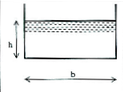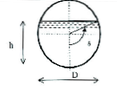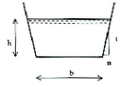# Section types

## Rectangular sectionThe rectangular section is characterized by the following parameters:

• width at bottom $$L$$ (in m)

## Circular sectionThe circular section is characterized by the following parameters:

• the pipe diameter $$D$$ (in m)
• the angle $$\theta$$ between the pipe bottom and the junction point between water surface and pipe (in Rad)

$$\theta = \arccos\left(1-\frac{y}{D/2}\right)$$

$$\theta' = \frac{2}{D\sqrt{1-\left(1-\frac{2y}{D}\right)^2}}$$

## Trapezoidal sectionThe trapezoidal section is characterized by the following parameters:

• width at bottom $$L$$ (in m)
• bank slope (inclination to the vertical: widening between the top and bottom of the slope divided by the depth.) $$m$$ (in m/m)

## Parabolic section

The parabolic section is characterized by a mirror width that can be expressed in the form:

$$B = \Lambda.y^k$$.

With $$k$$: coefficient between 0 and 1. $$k=0.5$$ corresponds to the true parabolic form.

$$\Lambda$$ can be calculated by giving:

• $$y_b$$: bank height (in m)
• $$B_b$$: embankment width (in m)

We then have: $$\Lambda = \frac{B_b}{y_b^k}$$